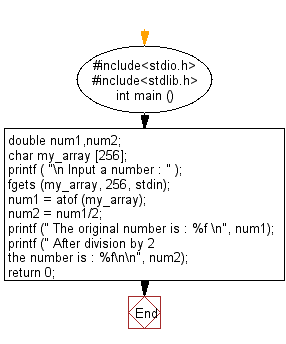﻿ C Program: Convert a string to a double - w3resource# C Exercises: Convert a string to a double

## C Variable Type : Exercise-14 with Solution

Write a C program to convert a string to a double.

Sample Solution:

C Code:

``````#include<stdio.h>
#include<stdlib.h>

int main ()
{
double num1,num2;
char my_array ;
printf ( "\n Input a number : " );
fgets (my_array, 256, stdin);
num1 = atof (my_array);
num2 = num1/2;
printf (" The original number is : %f \n", num1);
printf (" After division by 2 the number is : %f\n\n", num2);
return 0;
}
```
```

Sample Output:

```Input a number : 25
The original number is : 25.000000
After division by 2 the number is : 12.500000
```

Flowchart:C Programming Code Editor:

Improve this sample solution and post your code through Disqus

What is the difficulty level of this exercise?

Test your Programming skills with w3resource's quiz.

﻿

## C Programming: Tips of the Day

How I can print to stderr in C?

The syntax is almost the same as printf. With printf you give the string format and its contents i.e.:

`printf("my %s has %d chars\n", "string format", 30);`

With fprintf it is the same, except now you are also specifying the place to print to:

```File *myFile;
...
fprintf( myFile, "my %s has %d chars\n", "string format", 30);```

`fprintf( stderr, "my %s has %d chars\n", "string format", 30);`